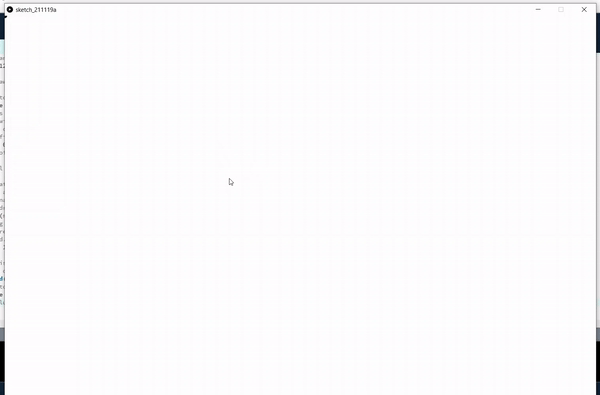# How to create a simple drawing board in Processing with Python Mode?

• Last Updated : 17 May, 2022

Processing is a programming language, and a development environment. It is an open-source software to create visual arts, graphics and animation using programming. It supports around 8 different modes, and in this article, we will be using Python Mode.

In this article, we are going to create a simple drawing board using Processing with Python Mode. In case if you have not installed the Processing Software yet, follow this article to Download and Install Processing, and Setup Python Mode.

### Approach:

• Firstly open Processing software and choose Python Mode.
• Then in the coding part, we are going to define three functions, setup(), draw(), and mouseDragged()
• setup() function is called before the start of the program. Here we have to set the background color and size of the window.
• In the mouseDragged() function we are simply going to assign 0 to the global variable value. This function is called when the mouse is being dragged i.e. when the user clicks and holds and moves the mouse button.
• draw() method is called throughout the program execution. In this method, we are going to check if the value is 0, (i.e if mouse is being dragged). If it is true, then we are going to draw a circle (using ellipse() function) at the position of the cursor. fill(0) is used to fill the circle with black color. We can get X and Y coordinates of the cursor using the variables mouseX and mouseY. Once the circle is drawn, set the value to 1 (i.e. circle is drawn for the current position of the mouse).
• Whenever the user drags the mouse, the if condition in the draw method turns true, and draws circle repeatedly throughout the movement of the cursor thus creating a drawing effect.

Below is the implementation:

Here is the Python code for the above approach. Now type the following code in the code editor.

## Python3

 `# global variable``value ``=` `1`` ` `# function to setup size of``# output window``def` `setup():`` ` `    ``# to set background color of window``    ``# to white color``    ``background(``255``)`` ` `    ``# to set width and height of window``    ``# to 1500px and 1200px respectively``    ``size(``1500``, ``1200``)`` ` `# function to draw on the window``def` `draw():`` ` `    ``# referring to the global value``    ``global` `value`` ` `    ``# if mouse is dragged then``    ``# the value will be set to 0``    ``# so here by checking if value equal to 0,``    ``# we are confirming that the mouse is being``    ``# dragged``    ``if` `value ``=``=` `0``:`` ` `        ``# width of circle``        ``r ``=` `10`` ` `        ``# to fill the color of circle to black``        ``fill(``0``)`` ` `        ``# to create a circle at the position of``        ``# mouse clicked mouseX and mouseY coordinates``        ``# represents x and y coordinates of mouse``        ``# respectively when it is being dragged.``        ``ellipse(mouseX, mouseY, r, r)`` ` `        ``# setting value to 1, which means a circle``        ``# is drawn at current position and waiting``        ``# for the mouse to be dragged.``        ``value ``=` `1`` ` `# this function is called when``# mouse is being dragged (mouse click+ hold + move)``def` `mouseDragged():`` ` `    ``# referring to global value``    ``global` `value`` ` `    ``# setting value to 0``    ``value ``=` `0`

Output:Processing with Python Mode

My Personal Notes arrow_drop_up